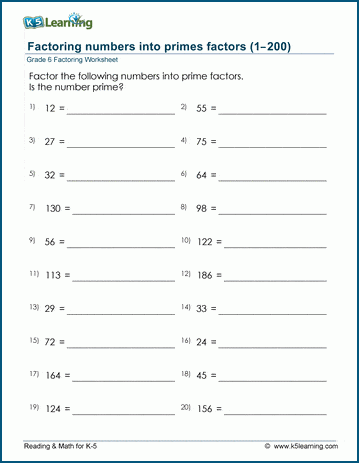# GCF and LCM Worksheets

## Grade 6 Factoring Worksheets: GCF & LCM

These math worksheets cover factoring to prime numbers as well as finding the greatest common factor (GCF) or least common multiple (LCM) of two numbers.

## Prime Factors

Prime factors (#'s < 100) 32: 2x2x2x2x2
Prime factors (#'s < 500) 214:  2 x 107

## Greatest common factors

Find the GCF of 2 numbers GCF of 21, 35 is 7
Find the GCF of 2 numbers (<100) GCF of 12, 100 is 4
Find the GCF of 3 numbers GCF of 6, 21, 84 is 3

## Least common multiples

Find the LCM of 2 numbers LCM of 3, 4 is 12
Find the LCM of 2 numbers (<50) LCM of 4, 34 is 68
Find the LCM of 3 numbers LCM of 4, 7, 14 is 28